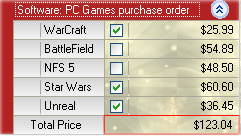20.2 (EAP/Beta)
20.1
19.2
19.1
18.2
18.1
17.2

# VGridControlBase.GetCellValue(MultiEditorRow, Int32, Int32) Method

Returns the value of the specified cell within the multi-editor row.

Namespace: DevExpress.XtraVerticalGrid

Assembly: DevExpress.XtraVerticalGrid.v20.1.dll

## Declaration

``````public virtual object GetCellValue(
MultiEditorRow meRow,
int recordIndex,
int cellIndex
)``````
``````Public Overridable Function GetCellValue(
meRow As MultiEditorRow,
recordIndex As Integer,
cellIndex As Integer
) As Object``````

## Parameters

Name Type Description
meRow MultiEditorRow

A MultiEditorRow object that represents the row where the cell resides.

recordIndex Int32

A zero-based integer that specifies the index of the record which contains the cell.

cellIndex Int32

A zero-based integer that specifies the cell's index.

## Returns

Type Description
Object

An object that represents the cell's value. null (Nothing in Visual Basic) if no cell is found.

## Examples

The following sample code handles the VGridControlBase.CellValueChanged event to sum up the prices of the selected games in the purchase order list. The image below shows the result.``````using DevExpress.XtraVerticalGrid;
using DevExpress.XtraVerticalGrid.Rows;
using DevExpress.XtraVerticalGrid.Events;

private void vGridControl1_CellValueChanged(object sender, CellValueChangedEventArgs e) {
double sum = 0;
BaseRow row = vGridControl1.GetFirst();
while (vGridControl1.GetNext(row) != null){
if (row.XtraRowTypeID == 2){
if (Convert.ToBoolean(vGridControl1.GetCellValue(row as MultiEditorRow, 0, 0)) == true)
sum += Convert.ToDouble(vGridControl1.GetCellValue(row as MultiEditorRow, 0, 1));
}
row = vGridControl1.GetNext(row);
}
vGridControl1.SetCellValue(rowPrice, 0, sum);
}
``````